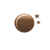# Convert 11 m to in (11 m to in)

 How to convert 11 m to in? meter: m inches: in
You may also interested in: meters to inches Converter / Convert 1 m to in

The online meters to inches Converter is used to convert the length from meters to inches.

#### The meter to inches Conversion Formula to convert 11 meters to inches

To know how many inches in a meter, you can use the following formula to convert from meter to inches :

X(inches) = y(m) ÷ 0.0254

To convert 11 m to in:

X(inches) = 11(m) ÷ 0.0254

#### meters to inches conversion table (Example: 11 m = 433.070866 in)

Meter (m) inch (in)
1 m 39.370079 in
2 m 78.740157 in
3 m 118.110236 in
4 m 157.480315 in
5 m 196.850394 in
6 m 236.220472 in
7 m 275.590551 in
8 m 314.96063 in
9 m 354.330709 in
10 m 393.700787 in
20 m 787.401575 in
30 m 1181.102362 in
40 m 1574.80315 in
50 m 1968.503937 in
60 m 2362.204724 in
70 m 2755.905512 in
80 m 3149.606299 in
90 m 3543.307087 in
100 m 3937.007874 in
500 m 19685.03937 in
1000 m 39370.07874 in

#### Frequently asked questions to convert meter to inches

How to convert 120 meters to inches ?

How to convert 76 meters to inches ?

How to convert 97 meters to inches ?

How to convert 92 meters to inches ?

How to convert 165 meters to inches ?

How to convert 152 meters to inches ?

#### Online Converter to convert meter to inches

We provide the online converter for free. You can use the meter to inches converter to convert the meter to inches.

#### References

More references for Metre and Inches

#### Best conversion unit for 11 meters

The best conversion unit defined in our website is to convert a number as the unit that is the lowest without going lower than 1. For 11 meters, the best unit to convert to is 11 meters.

#### Local Weather Status

Ashburn, US
21st January, 2020 Tuesday
Clear Sky-9 - -4.44
Humidity: 67 %
Wind: 3.6 km/h